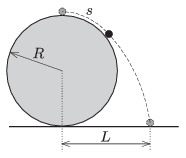Mathematical and Physical Journal
for High Schools
Issued by the MATFUND Foundation
 Already signed up? New to KöMaL?

#Problem P. 4653. (September 2014)

P. 4653. There is a small (point-like) object at the topmost point of a fixed sphere of absolutely smooth surface. If this object is slightly displaced from its equilibrium position it slides frictionlessly along the surface of the sphere for a while, and then leaving the sphere it falls down.$\displaystyle a)$ How much distance is covered by the small object along the surface of the sphere until it leaves the sphere?

$\displaystyle b)$ Measured from the vertical diameter of the sphere, at what distance $\displaystyle L$ will the object hit the horizontal surface?

The radius of the sphere is $\displaystyle R=1.5$ m.

(4 pont)

Deadline expired on October 10, 2014.

### Statistics:

 105 students sent a solution. 4 points: 73 students. 3 points: 14 students. 2 points: 9 students. 1 point: 2 students. 0 point: 7 students.

Problems in Physics of KöMaL, September 2014